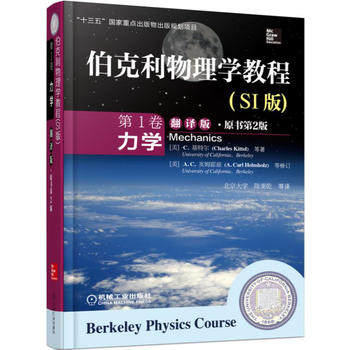## 耶鲁基础物理3.2牛顿第二定律$$\boldsymbol F=m\boldsymbol a \label{3.1}\tag{3.1}$$

- 阅读剩余部分 -

## 耶鲁基础物理3.3第二定律的两个应用\begin{equation} F=-kx \label{3.6}\tag{3.6} \end{equation}- 阅读剩余部分 -

## 长链落体《伯克利物理学教程·力学》翻译版封面

《伯克利物理学教程·力学》第6章习题8：

- 阅读剩余部分 -

## 匀速拉地毯最少需要多大的力？- 阅读剩余部分 -

## 大学物理测验1——运动学和牛顿运动定律

1. 一质点沿$x$轴运动，运动学方程为$x=t^3-3t^2$，问在哪些时间间隔内沿x轴正方向运动，哪些时间间隔内沿x轴负方向运动，哪些时间间隔内质点加速运动，哪些时间间隔内质点减速运动？

2. 一质点运动学方程为$x=t^2$，$y=(t-1)^2$，x和y均以m为单位，时间t以秒为单位，求质点的运动轨迹，求在$t=2\mathrm s$时，质点的速度和加速度。

3. 一质点运动学方程为$\vec{r}=2t\vec{i}+(2-t^2)\vec{j}$，$\vec{r}$和时间t分别以m和s为单位。求从$t=0$到$t=1\mathrm s$时间间隔内质点的位移。$t=1\mathrm s$时质点的速度和加速度。求质点的轨迹方程。

4. 一质点沿x轴运动，加速度a与位置x的关系为$a=-2x$，求速度v与位置x的关系。

5. 一质点沿半径为的圆周运动，沿圆弧的自然坐标为$s$。运动学方程为$s=\pi t^2+\pi t$。求质点绕行一周所经历的路程、位移、平均速度和平均速率。求第1秒末时的速度和加速度大小。

6. 一质点沿半径为$R=1\mathrm m$的圆周运动，用角坐标表示的运动学方程为$\theta=2+4t^3$，$\theta$的单位为rad，t但单位为s。求t=2s时，质点的加速度。何时质点加速度与半径夹角为45°？

7. 以质点在随时间变化的力$F=F_0(1-kt)$的作用下沿$x$轴运动，已知在$t=0$时刻，质点位于处$x_0=1\mathrm m$，速度$v_0=1\mathrm {m/s}$，求质点运动方程。

8. 以初速度$v_0$从地面竖直上抛一质量为$m$的小球，小球受空气黏滞阻力为$f=-\alpha mv^2$，$\alpha$为常数。请写出$\alpha$的量纲。当小球回到地面时，速度大小为多少？